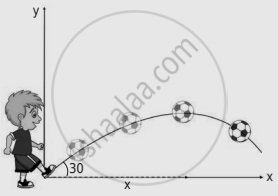Tamil Nadu Board of Secondary EducationHSC Science Class 11th

# A football player hits the ball with a speed of 20 m s-1 with an angle of 30° with respect to horizontal direction as shown in the figure. The goal post is at a distance of 40 m from him. - Physics

Sum

A football player hits the ball with a speed of 20 m s-1 with an angle of 30° with respect to horizontal direction as shown in the figure. The goal post is at a distance of 40 m from him. Find out whether the ball reaches the goal post?#### Solution

Given:

Initial speed (u) = 20 ms-1

The angle of projection (θ) = 30°

The distance of the goal post = 40 m

Solution:

Range of the projectile R = (u^2 sin2theta)/g = (400 xx sin 60^circ)/9.8 = (400 xx sqrt3/2)/9.8 = 35.35 m

The distance of the goal post is 40 m. But the range of the ball is 35.35 m only. So the ball will not reach the goal post.

Concept: Projectile Motion
Is there an error in this question or solution?

Share